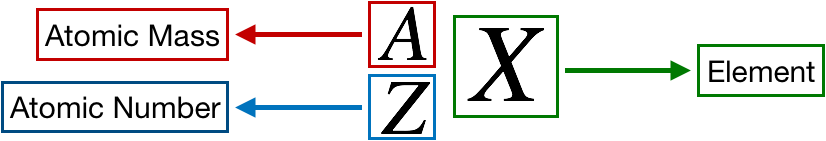# Problem: Fill in the gaps in the following table, assuming each column represents a neutral atom:Symbol79BrProtons2582Neutrons3064Electrons4886Mass no.222207Complete the fifth row of the table.

###### FREE Expert Solution

We’re being asked to determine the mass number and complete the fifth row of the given table.

Recall that the symbol for an element or ion is as follows:83% (326 ratings)###### Problem Details
Fill in the gaps in the following table, assuming each column represents a neutral atom:

 Symbol 79Br Protons 25 82 Neutrons 30 64 Electrons 48 86 Mass no. 222 207

Complete the fifth row of the table.

Frequently Asked Questions

What scientific concept do you need to know in order to solve this problem?

Our tutors have indicated that to solve this problem you will need to apply the Isotopes concept. You can view video lessons to learn Isotopes. Or if you need more Isotopes practice, you can also practice Isotopes practice problems.